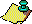Contents Next: Are there non-trivial Up: The 3x+1 problem. Previous: How many elements

Much less is known about the total stopping time function than about the stopping time function. One phenomenon immediately observable from a table of the total stopping times of small integers is the occurrence of many pairs and triples of integers having the same finite total stopping time. From Figure 1 we see that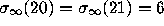,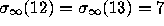,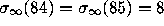,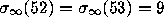, and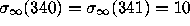. Indeed for larger values of n, multiple consecutive values occur with the same total stopping time. For example there are 17 consecutive values of n with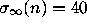for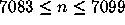. A related phenomenon is that over short ranges of n the function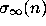tends to assume only a few values (C. W. Dodge ). As an example the values offor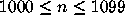are given in Table 3. Only 19 values forare observed, for which a frequency count is given in Table 4. Both of these phenomena have a simple explanation; they are caused by coalescence of trajectories of different n's after a few steps. For example the trajectories of 8k + 4 and 8k + 5 coalesce after 3 steps, for all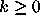. More generally, the large number of coalescences of numbers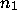and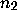close together in size can be traced to the trivial cycle (1,2), as follows. Supposeandhave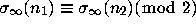, and let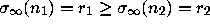. Then the trajectories ofandcoalesce after at most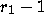iterations, since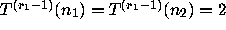, since the trajectory ofcontinues to cycle around the trivial cycle. If in addition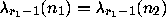, which nearly always happens ifandare about the same size, then the trajectories of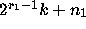, and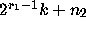coalesce after at mostiterations, for. In particular,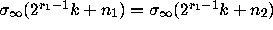then holds for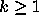. In this case the original coalescence ofandhas produced an infinite arithmetic progression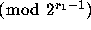of coalescences. The gradual accumulation of all these arithmetic progressions of coalescences of numbers close together in size leads to the phenomena observed in Tables 3 and 4.

Although the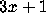Conjecture asserts that all integers n have a finite total stopping time, the strongest result proved so far concerning the density of the set of integers with a finite total stopping time is much weaker.

TABLE 3. Values of the total stopping timefor.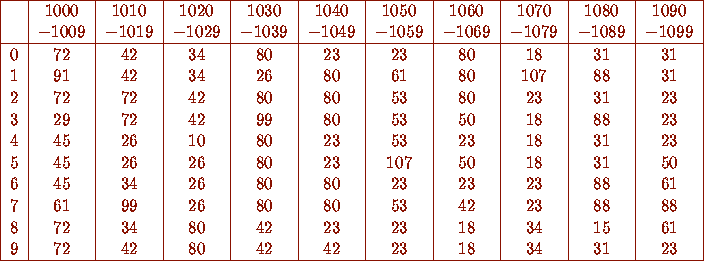TABLE 4. Values ofand their frequencies for.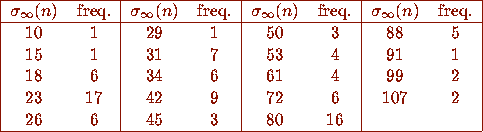### Theorem G

(Crandall). Let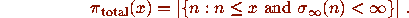Then there is a positive constant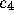such that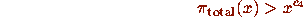for all sufficiently large x. (Click here for the current best result.)

Assuming that theConjecture is true, one can consider the problem of determining the expected size of the total stopping time function. Crandall  and Shanks  were guided by probabilistic heuristic arguments (like the one described earlier) to conjecture that the average order ofshould be a constant times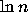; more precisely, that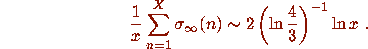( Click here and here for further information on.) A modest amount of empirical evidence supports these conjectures, see .Contents Next: Are there non-trivial Up: The 3x+1 problem. Previous: How many elements